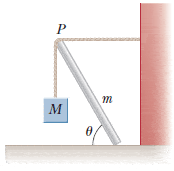# Statics problem

## Homework StatementA uniform beam of mass m is inclined at an angle θ to the horizontal. Its upper end (point P) produces a 90° bend in a very rough rope tied to a wall, and its lower end rests on a rough floor (see figure below). Let μs represent the coefficient of static friction between beam and floor. Assume μs is less than the cotangent of θ.
(a) Find an expression for the maximum mass M that can be suspended from the top before the beam slips. (Use any variable or symbol stated above along with the following as necessary: g.)
(b) Determine the magnitude of the reaction force (R) at the floor in terms of m, M, g, and μs.
(c) Determine the magnitude of the force (F) exerted by the beam on the rope at P in terms of m, M, g, and μs.

Net torque = 0
Fnet = 0

## The Attempt at a Solution

I have no clue where to start. Previous ones were easier, I just need to get started.